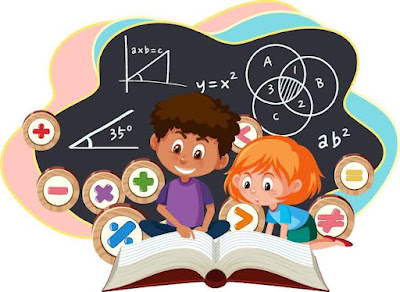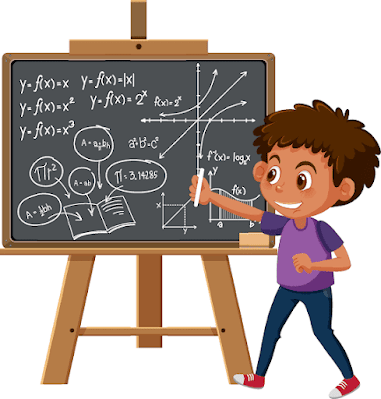## Quote of The Day

Selepas musim yang berganti, cara terbaik untuk memudahkan syukurmu terlantun adalah dengan menyederhanakan harapanmu hari ini.

## Jumat, 02 Desember 2022

### Tips and Strategies to Win the Math Olympiad

Study These Strategies to Win the Math Olympiad

If you want to win the math Olympiad, you need to study these strategies! In this article, we will discuss some of the most effective ways to score high in the competition. Whether you are a beginner or an experienced math student, these tips can help you achieve your goals.

## Understand the basics of the math Olympiad.

The math Olympiad is a challenging competition that requires strong math skills. In order to compete well, students must be prepared for rigorous problems. To do well in the math Olympiad, students need to study for it and practice problem-solving skills.

The math Olympiad is a difficult competition, and it helps to have a basic understanding of the procedures and concepts involved. In order to compete successfully, students must first be familiar with the basics of the competition: how the rounds are conducted, what is required of them on each round, and so on.

To win in the math Olympiad, it is important to be prepared for the challenge. The best way to prepare is to study regularly and to memorize the math formulas. In addition, it is also important to practice problem-solving skills often. Following some strategies that are used by the experts can help you succeed.How to win math olympiade

## Make a study schedule.

To be successful in the math Olympiad, you need to make a study schedule that works for you. There are many different types of schedules, and what works for one student might not work for another. However, there are some general tips that can help you create a schedule that will help you achieve your goals.

First, make sure that your study time is scheduled in such a way that you have enough time to review all the material and practice the problems.

Second, try to break up your study time into small, manageable chunks. This will help you focus and avoid feeling overwhelmed.

And finally, make sure that you take regular breaks so that you don’t get too tense or fatigued.

Memorizing math formulas can be one of the most important ways to improve your chances of winning in the math Olympiad. Once students have memorized the formulas, they can start to solve problems more easily.

One way to help students memorize formulas is to make a study schedule. Once students know when and where they will be studying, it becomes much easier to focus. It is also helpful to break down the math Olympiad competition into smaller parts, so that the formulas become more manageable.

Another strategy for memorizing formulas is to practice problem-solving skills. By solving problems, students can develop their mental prowess and muscle memory in a controlled setting. This will make it easier for them to solve problems on the actual math exam.

While memorization and problem-solving skills are two of the most important ways to win in the math Olympiad, it is also important for students to have a study plan in place. By following a study plan, students can avoid feeling overwhelmed by the competition and focus on studying the material that is most important.

Planning and organizing your time can help you focus on the math Olympiad and learn as much as possible. By scheduling your studying in advance, you can avoid unnecessary distractions and make sure that you are getting the most out of your time in the library or at home.Learn and practice math formula to win math olympiade

## Memorize math formulas.

Learning and using math formulas will help you solve problems more efficiently. When solving a problem, it is often helpful to know the order of operations: Parentheses, Exponents, Multiplication and Division (from left to right), Addition and Subtraction (from left to right). Memorizing these rules can help you solve problems quickly and easily.

There are many different ways to memorize math formulas. Some people prefer to create flashcards, learn them by heart, or write them down mentally as they study them. Which method is best for you depends on your individual learning style. However, all of these methods work well in the long run if you practice regularly.

## Practice problem-solving skills.

One of the most important skills to develop for winning in the math Olympiad is problem-solving ability. While it can seem daunting at first, following a step-by-step process and using tried and true methods will help you solve problems quickly and efficiently.

When trying to solve a math problem, always try to simplify the equation before tackling it. By doing this, you can identify whatever factors are causing the equation to be difficult to solve, and you can work on resolving them one by one.

If a problem requires solving two separate equations, be sure to use parentheses and brackets to clearly indicate the order in which you are working on them. Additionally, make sure to write down everything you are doing in order to remember how each step resolves the problem.

Avoid making assumptions about the answer to a problem - instead, test different hypotheses until you find the correct one. This will help you avoid getting stuck with an incorrect answer, and it will also allow you to see how different factors interact with each other.

Be patient when attempting to solve math problems - sometimes they take a long time to hatch an answer. However, by following these tips, you will be well on your way to becoming a math Olympiad champion!

The math Olympiad is a rigorous competition that requires students to be extremely well-prepared. To be a successful competitor, you need to understand the basics of the competition and make a study schedule accordingly. You also need to practice problem solving skills and memorize math formulas. Following strategies that are effective for you will help you win. Stay positive and have fun while you compete!

Practice solving difficult problems before competing in the math Olympiad. The best way to improve your problem-solving skills is to practice solving problems that are similar to those that you will face during the competition. Furthermore, it’s important to remember that problem-solving does not always require a perfect answer. Often, solving a problem simply involves finding a solution that works.

## Follow strategies the experts use.

Following the strategies of successful math Olympiad participants can lead to improved results.

Many top competitors follow a “problem-based learning” approach, which means that they focus on solving real-world problems rather than relying solely on mathematical formulas.

By solving problems in this way, students develop practical math skills that they can use throughout their academic career.

### Here are five tips for success that they use:

1. Make a study schedule.

2. Memorize math formulas.

3. Practice problem-solving skills.

4. Follow strategies the experts use.

5. Take online courses and subscribe to math newsletters.